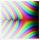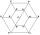Electronics: Resistors in parallel

From relation for calculating the resistance of parallel combination of resistors:Calculate the R, if R1 = 2Ω a R2 = 15Ω

Result

R =  1.76 Ω

Solution:Leave us a comment of example and its solution (i.e. if it is still somewhat unclear...):Be the first to comment!To solve this example are needed these knowledge from mathematics:

Looking for help with calculating harmonic mean? Looking for a statistical calculator?

Next similar examples:

1. Theorem proveWe want to prove the sentence: If the natural number n is divisible by six, then n is divisible by three. From what assumption we started?
2. ResistanceA resistor having an electrical resistance of 1.5 k ohms passes an electrical current of 0.1 A. Calculate what voltage is between the terminals of the resistor.
3. Volleyball8 girls wants to play volleyball against boys. On the field at one time can be six players per team. How many initial teams of this girls may trainer to choose?
4. ArcConvert to arc measures (radians): 924 ° Result write as a multiple of π
5. ReciprocalWhat is the reciprocal of the sum of the reciprocals of 4 and 9?
6. 6 termsFind the first six terms of the sequence. a1 = 7, an = an-1 + 6
7. One halfOne half of ? is: ?
8. TreesA certain species of tree grows an average of 0.5 cm per week. Write an equation for the sequence that represents the weekly height of this tree in centimeters if the measurements begin when the tree is 200 centimeters tall.
9. PowersExpress the expression ? as the n-th power of the base 10.
10. Two equationsSolve equations (use adding and subtracting of linear equations): -4x+11y=5 6x-11y=-5
11. Linear systemSolve a set of two equations of two unknowns: 1.5x+1.2y=0.6 0.8x-0.2y=2
12. Functions f,gFind g(1) if g(x) = 3x - x2 Find f(5) if f(x) = x + 1/2
13. Nineteenth memberFind the nineteenth member of the arithmetic sequence: a1=33 d=5 find a19
14. Factory and divisionsThe factory consists of three auxiliary divisions total 2,406 employees. The second division has 76 employees less than 1st division and 3rd division has 212 employees more than the 2nd. How many employees has each division?
15. TrigonometryIs true equality? ?
16. Hexagon = 8 partsDivide the regular hexagon into eight equal parts.
17. SequenceWrite the first 6 members of these sequence: a1 = 5 a2 = 7 an+2 = an+1 +2 an